# Compound interest and powers

## 2007-02-11

In some of my mathematical calculations, I end up computing an expression that involves a number very close to one raised to a very large power. This term can often be approximated by an exponential function, but I can never quite remember the relationship. An example involving compound interest may help me remember better in the future.

If you invest an amount of money A for t years at a simple interest rate, i, the amount of money that you earn is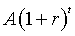and if you compound the interest n times per year, the amount you earn isIf you let n go to infinity, this term converges toThis formula is well known to people in finance and in mathematics. A nice explanation can be found in the Wikipedia article on compound interest.

As an example of how I would use this, I had an expression of the form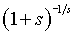What would this equal for values of s close to zero? Replace s with 1/n to get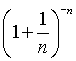This is just like the compound interest formula with A=1, r=1, and t=-1. A purist might quibble that compound interest for a negative amount of time makes no sense, but there is indeed an interpretation for this in a financial context. Even if there were no such interpretation, the mathematical relationship holds anyway.

The limit as n approaches infinity (which is equivalent to the limit as s approaches zero) is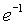How good is this approximation? The constant e is 2.718 and the ratio 1/e is 0.3679. For s=0.1, you getFor s=0.01, you get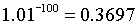For s=0.001, you getYou can find an earlier version of this page on my old website.